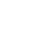发布# MATLAB

Matlab论坛，Matlab软件的问答讨论，以及视频教程、实例教学等学习资料分享。

## 问答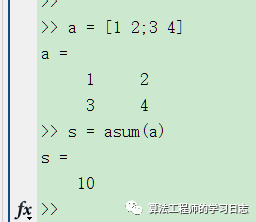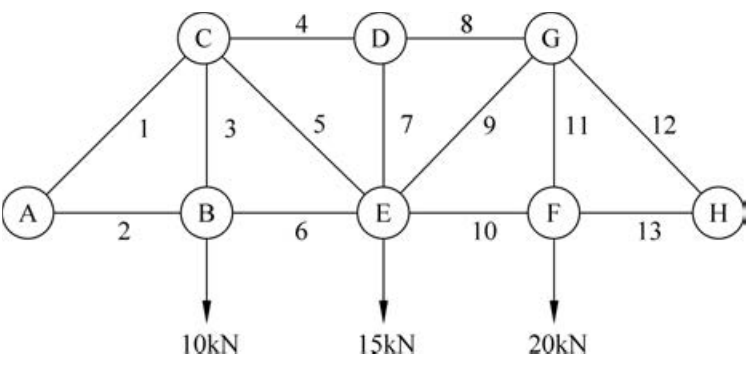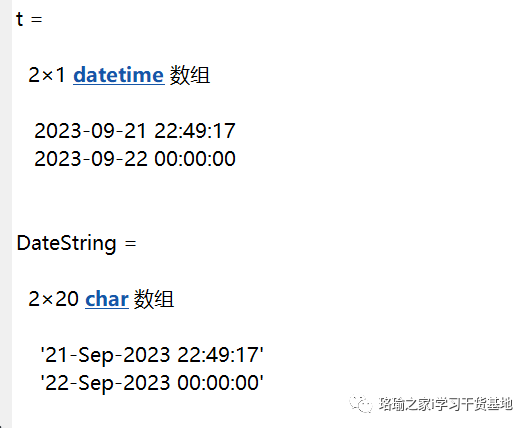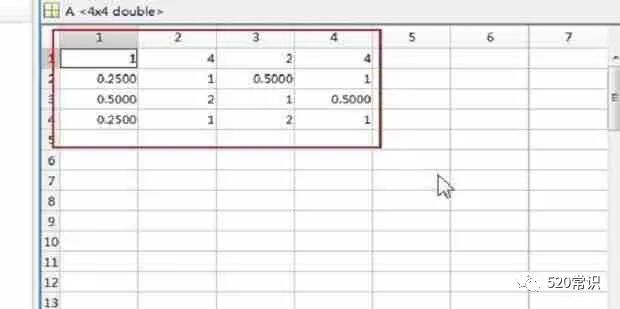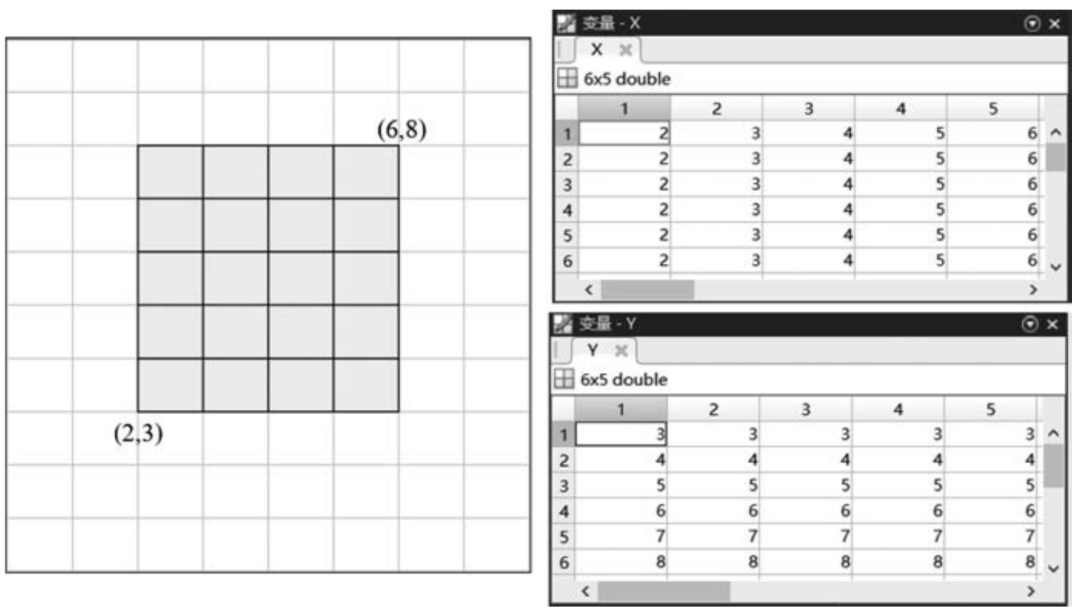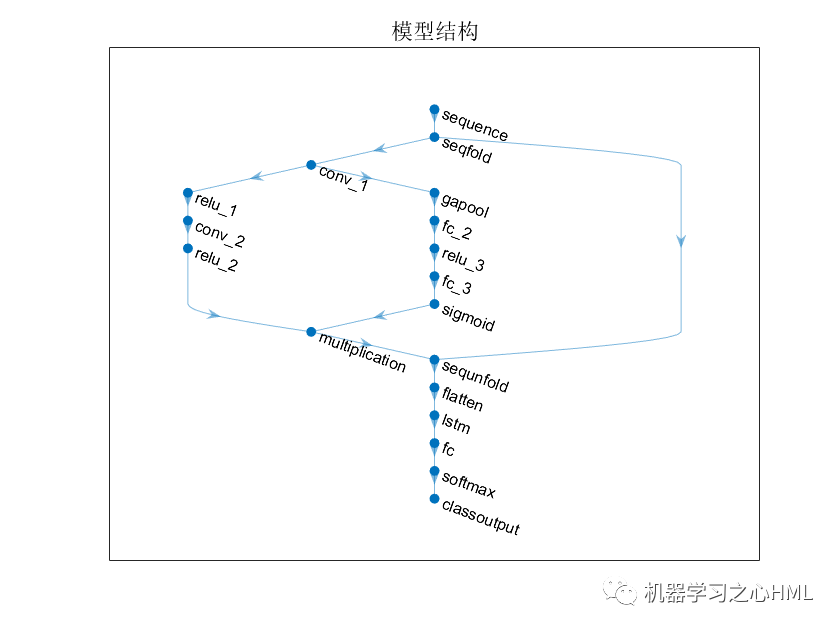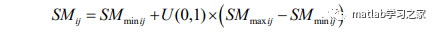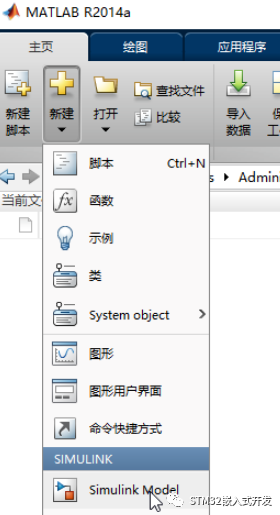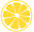fclose all; close all; clear; clc; %% Define functions and parameters syms x; f = 0.1*(x^2 + 2*x + 1); diff_f = diff(f,x); n_max = 100; e_tol = 1e-5; x_0 = 5; f_0 = subs(f,x,x_0); error = 10*e_tol; t11月1年全部

1月1年总榜
项目客服
培训客服
平台客服
顶部